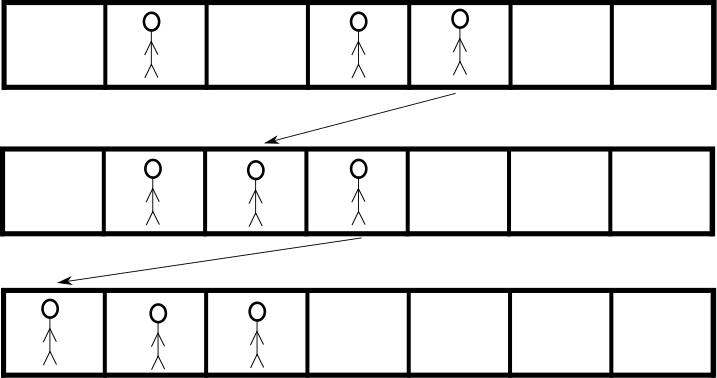시간 제한 메모리 제한 제출 정답 맞은 사람 정답 비율
2 초 1024 MB 0 0 0 0.000%

## 문제

$N$ friends are playing a game. The game is played on a row of $L$ squares, numbered from $0$ to $L - 1$, where squares $i$ and $i+1$ are adjacent to each other. At most one friend stand on each square at any given time. In each step of the game, one friend jumps from its current square to a new (non-occupied) square.

In any moment of the game, the score of a friend is the length of the longest contiguous segment of friends it is part of. This means that if a friend stands on some position $x$, and there are friends on positions $a, a + 1, ..., x - 1, x, x + 1, ..., b - 1, b$, the score of the friend is $b - a + 1$.

The total score of the game is the sum of scores for all friends. At various times during the game, the friends wonder what their current total score is.

## 예제

Assume that we have $N = 3$ friends playing on a strip of length $L = 7$. Initially, their locations are $1, 3, 4$. The score of the friend on location 1 is just 1, since it has no friends to the left or to the right. However, the friends at locations 3 and 4 make a segment of length 2, so each of their scores are 2. The score of the game is thus $1 + 2 + 2 = 5$.

The first jump is from position $4$ to $2$, making the new positions $1, 2, 3$. Here, all friends make up a segment of length 3, so each of the friends have score 3, making the total score 9.

The second and final jump is from $3$ to $0$, resulting in the positions $0, 1, 2$. All friends still make up a segment of length 3, so the score is unchanged from last time, i.e. it is still 9.Figure 1: Illustration of the example

## 구현

You will get all the jumps in the game, one by one. At some points, the friends will ask what the current score is. Your task is to implement the functions init(N, L, P), jump(A, B), and score():

• init(N, L, P) - this function will be called exactly once by the judge, at the start of the game.
• N: the number of friends playing.
• L: the number of locations the game is being played on.
• P: an array with $N$ elements. P[i] ($0 \le i < N$) contains the original position of the $i$:th friend.
• The function has no return value.
• jump(A, B) - this function will be called once for every jump, in the order they are made.
• A: the position a friend is jumping from ($0 \le A < L$). This position will always contain a friend at the time of the jump.
• B: the position a friend is jumping to ($0 \le B < L$). This position will never contain a friend at the time of the jump.
• The function has no return value.
• score() - this function will be called when the friends wish to know their current score.
• The function should return the current score in the game.

A code skeleton containing the functions to be implemented, together with a sample grader, can be found at attachments.zip.

## 입력

The sample judge reads input in the following format:

• line $1$: N L Q
• line $2$: P P .. P[N - 1]
• lines $3$ to $3 + Q - 1$: each line represents either a jump or a score question. If the line is 0 A B, a jump from $A$ to $B$ is to be made, and if the line is 1 a scoring question is to be made.

## 출력

For each scoring question, the judge writes a line with the return value of score().

## 제한

• $1 \le N \le 100\,000$
• $1 \le L \le 10^9$
• $S + J \le 200\,000$

## 예제 입력 1

3 7 5
1 3 4
1
0 4 2
1
0 3 0
1


## 예제 출력 1

5
9
9


## 출처• 문제를 만든 사람: Johan Sannemo

## 제출할 수 있는 언어

C++17, Java 8, C++14, Java 8 (OpenJDK), Java 11, C++20, Java 15, C++14 (Clang), C++17 (Clang), C++20 (Clang)

## 채점 및 기타 정보

• 예제는 채점하지 않는다.